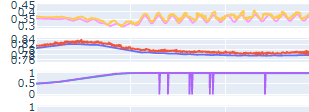# Efficient Time Distributed Dense

Let’s see if anyone can help me with this particular case. I have an input of dimension 1600x240 (1600 time steps and 240 features for each time step) and I want to apply a linear layer independently for each time step. I know that the pytorch nn.Linear module works with 2 dimensional inputs, but it doesn’t do exactly what I want. If I apply nn.Linear(240,100) on the input, we are only considering a 240x100 weight matrix. I have tried these four alternatives:

``````import torch.nn as nn

class Linear_only(nn.Module):
def __init__(self,):
super(Linear_only, self).__init__()
self.linear = nn.Linear(240,100)
def forward(self,x):
return self.linear(x)

class TimeDistributedLinear(nn.Module):
def __init__(self,):
super(TimeDistributedLinear, self).__init__()
self.linear = nn.Linear(240,100)
def forward(self,x):
x = x.view(-1,240)
return self.linear(x)

class UsingModulelist(nn.Module):
def __init__(self,):
super(UsingModulelist, self).__init__()
self.modulelistLinear = nn.ModuleList([nn.Linear(240,100) for i in range(1600)])
def forward(self,x):
output_list = []
for i in range(1600):
linear = self.modulelistLinear[i]
x_i = linear(x[:,i,:].view(16,240))
output_list.append(x_i)
x = torch.stack(output_list)
x = x.permute(1,0,2)
return x

class UsingParameter(nn.Module):
def __init__(self,):
super(UsingParameter, self).__init__()
self.weight_last_linear = nn.Parameter(torch.empty((1600, 240,100), requires_grad = True))

stdv = 1. / math.sqrt(self.weight_last_linear.size(1))
self.weight_last_linear.data.uniform_(-stdv, stdv)
if self.bias_last_linear is not None:
self.bias_last_linear.data.uniform_(-stdv, stdv)

def forward(self,x):
x = torch.einsum('ijl, jlm -> ijm',x,self.weight_last_linear)+self.bias_last_linear
x = x.view(16,1600,100)
return x

batch_size = 16
x = torch.rand(batch_size,1600, 240)
net_linear = Linear_only()
print(net_linear(x).size()) #torch.Size([16, 1600, 100]) Total Trainable Params: 24100
net_td_linear = TimeDistributedLinear()
print(net_td_linear(x).size()) #torch.Size([16, 1600, 100]) Total Trainable Params: 24100
net_modulelist = UsingModulelist()
print(net_modulelist(x).size()) #torch.Size([16, 1600, 100]) Total Trainable Params: 38560000
net_parameter = UsingParameter()
print(net_parameter(x).size()) #torch.Size([16, 1600, 100]) Total Trainable Params: 38560000
``````

Of these alternatives, the first two do not do what I want. The third one does exactly what I want, but the 1600 loop makes it take too long, so I’m not interested. And the last one, I think it should do what I really want but it doesn’t work properly, so I prefer not to use nn.Parameter(). Can you think of any other way?

Can you elaborate more on why doesn’t it work properly?

Hi @ID56,
I can’t give much information about the use case I am working on but it is a time-series anomaly detection case. The code I have attached is just a small part of a model that returns the reconstructions of the input time-series. When I use nn.Parameter(), I get strange spikes in the reconstructions, like these (in the lilac-colored series):Something that with nn.Linear() does not happen…